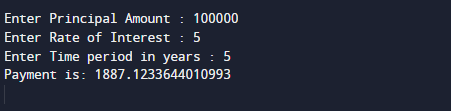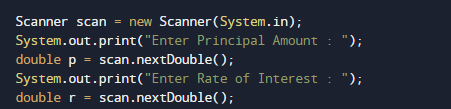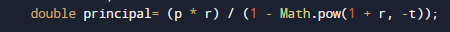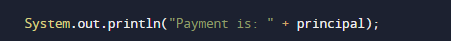# Create Simple Mortgage Calculator In Java

In this tutorial you will learn about the Create Simple Mortgage Calculator In Java and its application with practical example.

In this tutorial, we will learn to create a Java Program to Create Simple Mortgage Calculator using Java programming.

## Prerequisites

Before starting with this tutorial we assume that you are best aware of the following Java programming topics:

• Java Operators.
• Basic Input and Output function in Java.
• Class and Object in Java.
• Basic Java programming.
• If-else statements in Java.
• For loop in Java.

## Mortgage Payment

Know as the amount of money that you need to return to the person,where you have your property mortgaged.

Formula to calculate Mortgage is:

“M = P [{r(1+r)^n}/{(1+r)^n – 1}]”

where
M = Monthly payment
P = principal
r = rate
n = number of payments

## Create Simple Mortgage Calculator In Java

In this our program we will create a simple Mortgage Calculator. We would first declared and initialized the required variables. Next, we would prompt user to input value.Later in the program we will find Mortgage.

## OutputIn the above program, we have first declared and initialized a set variables required in the program.

• p = it will hold value of principal
• r= it will hold value of rate
• t= it will hold time in a year.
• principal= calculate payment of month.

In the next statement user will be prompted to enter the values of p,r and and later we will fine mortgage.Here we will to use following formula “principal= (p * r) / (1 – Math.pow(1 + r, -t))” and Math.pow() function which is define in Java standard library.it will take two parameter as a arguments p,r and t values supply to it.After calculating Mortgage value will be stored in  variable principal.Finally we will print the Mortgage of given values.

In this tutorial we have learn about the Create Simple Mortgage Calculator In Java and its application with practical example. I hope you will like this tutorial.